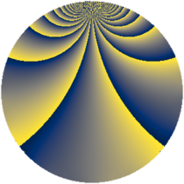# Properties

 Label 1815.2.rLevel $1815$ Weight $2$ Character orbit 1815.r Rep. character $\chi_{1815}(239,\cdot)$ Character field $\Q(\zeta_{10})$ Dimension $800$ Sturm bound $528$

# Related objects

## Defining parameters

 Level: $$N$$ $$=$$ $$1815 = 3 \cdot 5 \cdot 11^{2}$$ Weight: $$k$$ $$=$$ $$2$$ Character orbit: $$[\chi]$$ $$=$$ 1815.r (of order $$10$$ and degree $$4$$) Character conductor: $$\operatorname{cond}(\chi)$$ $$=$$ $$165$$ Character field: $$\Q(\zeta_{10})$$ Sturm bound: $$528$$

## Dimensions

The following table gives the dimensions of various subspaces of $$M_{2}(1815, [\chi])$$.

Total New Old
Modular forms 1152 928 224
Cusp forms 960 800 160
Eisenstein series 192 128 64

## Trace form

 $$800q + 196q^{4} + 10q^{6} + 8q^{9} + O(q^{10})$$ $$800q + 196q^{4} + 10q^{6} + 8q^{9} + 7q^{15} - 100q^{16} + 20q^{19} + 30q^{24} - 12q^{25} - 10q^{30} + 8q^{31} - 104q^{34} + 6q^{36} + 50q^{39} - 60q^{40} + 88q^{45} + 40q^{46} - 172q^{49} - 30q^{51} - 28q^{60} + 8q^{64} - 60q^{69} + 26q^{70} - 29q^{75} + 56q^{81} - 140q^{84} + 30q^{85} - 120q^{90} - 64q^{91} - 60q^{94} + 70q^{96} + O(q^{100})$$

## Decomposition of $$S_{2}^{\mathrm{new}}(1815, [\chi])$$ into newform subspaces

The newforms in this space have not yet been added to the LMFDB.

## Decomposition of $$S_{2}^{\mathrm{old}}(1815, [\chi])$$ into lower level spaces

$$S_{2}^{\mathrm{old}}(1815, [\chi]) \cong$$ $$S_{2}^{\mathrm{new}}(165, [\chi])$$$$^{\oplus 2}$$